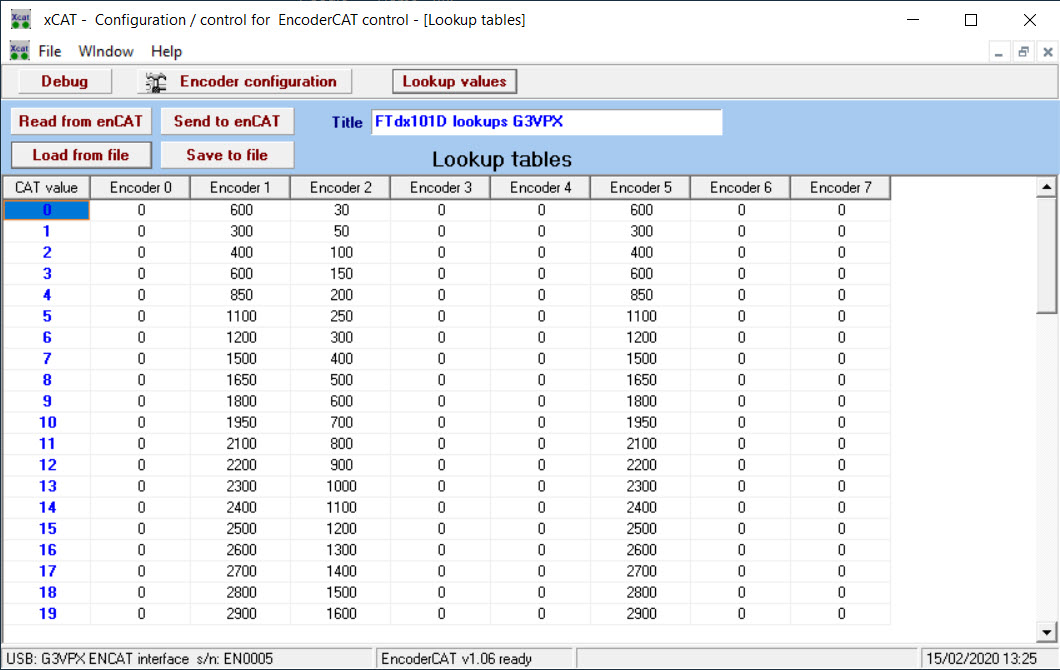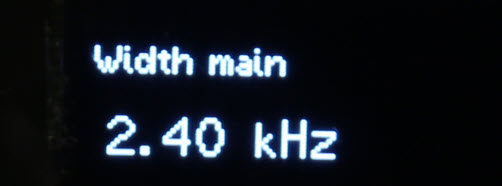﻿ xCAT - OLED display lookups

xCat   - Lookup list configuration

Example from Yaesu FTdx101D CAT manual:

Vox Delay Time:   CAT value range is 0 - 33

00 = 30msec     01 = 50 msec    02 = 100msec   03 = 150 msec  04 = 200msec   05 = 250msec

06 = 300msec   07 - 400msec    08 = 500msec   09 = 600msec ........  33 = 3000msec

It is observed that CAT value has a non-linear relationship to delay time:

A lookup table is needed ... .this is purely for the OLED display....   The actual CAT value is managed as 0 - 33.

Click the Lookup values button to display the window below:

Vox delay is encoder 2.A column of lookup values can be assigned to each of encoders 0 -7.
Each encoder can have up to 54 values.  Unused values outside the CAT value range are ignored.

If the lookup field is checked in encoder configuration then the OLED displayed value will be from the lookup column.

The lookup values are edited directly on the grid.

The lookup values are stored in EncoderCAT processor EEPROM and in disc file.
This is managed as with encoder configuration data with buttons:
 Please note that the order of events is lookup  >  scaling > decimal point.Thus the mult, div and  decp settings can be applied to the lookup values. eg:   Encoder 5 in the above example controls main receiver IF passband width. I entered lookup values in Hz. However, If we set div = 10 then 2400 becomes 240. Then, if we set decp = 2 and units = 'kHz', this will display as 2.40 kHz.